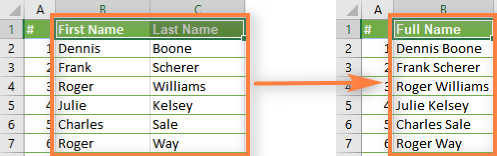## Find the equation of a line on Excel – By KulfiSoftware

Excel has four ways to find the equation of a line on excel: LINEST (or LONGEST) function, trendline on a chart, regression analysis in the Data…Analysis ToolPak, and Solver. Each of these methods uses the least squares method to find the coefficients of the equation.

I suggest looking at the R-squared statistic when you do this to make sure the equation is explaining most of the variation ...## Why is Excel such an undervalued tool for data analysis?

### Reasons for why is excel an undervalued tool for data analysis is: by kulfisoftware

Excel is used to do data analysis in a convenient and easy way with the shortest time required.

• Most of the people compare excel, with the highly recommended tools and they attained excel as useful. Excel such an undervalued tool for data analysis.

### It’s ...## Mark Or Highlight Word In An Excel File:

Today, we will show you how to mark or highlight word in an Excel File. Highlight words in an excel file are very easy as it looks, some of them think that highlighting or marking an excel file is hard but NO! Kulfisoftware will show you the easiest and quickest way to highlight words in an excel file. So, Stay Tuned!

A spreadsheet is a special way of organizing data into rows and columns to make it simpler to read ...## Why is Microsoft Excel not a database?Why is Microsoft Excel not a database? the question arises. So here are some of the reasons for why is EXCEL is not a database. by kulfisoftware.

The simplest thing you can do is add formulas that refer to the cells in sheet 1 containing the information you want to replicate. So a simple =Sheet1!A1 should be enough. Repeat for ...## What is the method to collapse or expand rows & columns in excel sheet?

The simple method to collapse or expand rows & columns in excel sheet are as follows:

## Here is a quick step-by-step example to show you how.

Here are the basic steps:

• First, you have to group the row/column selection
• THEN, you can collapse or expand that group

## Here is how you group rows or columns and then expand/collapse the group:

• First, you need to group the columns/rows.

• To group the ...## How can I automatically duplicate data from Excel sheet to another?

To automatically duplicate data from Excel sheet to another. The simplest thing you can do is add formulas that refer to the cells in sheet 1 containing the information you want to replicate. So a simple =Sheet1!A1 should be enough. Repeat for as many cells as necessary across the row and copy down as required. Like that every time you add a new record the information is replicated/linked ...## STEPS:

If you’re only combining two cells, the ampersand “&” is the easiest way – as mentioned by Vitor Barreto, but if you’re combining more than two there are a few functions you could use.If you’re using the latest version of Excel, try out the TEXT ...## What do you need to know to be considered advanced in excel?

Need to know to be considered advanced in excel. Excel does a lot and most training companies and trainers break up Excel into three levels: Basic, Intermediate, and Advanced. Here is what I would say makes you advanced. This list is not all-inclusive:

After surpassing main formatting features in Excel, you will come across Excel formulas & functions part, which is the major feature of Microsoft Excel. ...## How do you split a cell into multiple rows in Excel?

Split a cell into multiple rows in Excel. An easy way to do this would be to use the “text to columns” feature. Let’s learn through an example!

Tackling this task is a two-step process.

Excel is an electronic spreadsheet program.

An electronic spreadsheet is a computer software program that is used for storing, organizing and manipulating data.

## In our example, we will ...## Is learning VBA Excel worth all the efforts?

Our today’s question is learning VBA Excel worth all the efforts. Read the below article and find your answer.(Likely) no.

1. Microsoft no longer supports VBA: Since 2010, Microsoft no longer supports VBA and has tried (and failed) to remove and replace VBA from Microsoft Office.
2. Popularity: VBA has been in decline for years and replaced by far superior tools and languages ...
Page 2 of 6 12345...»